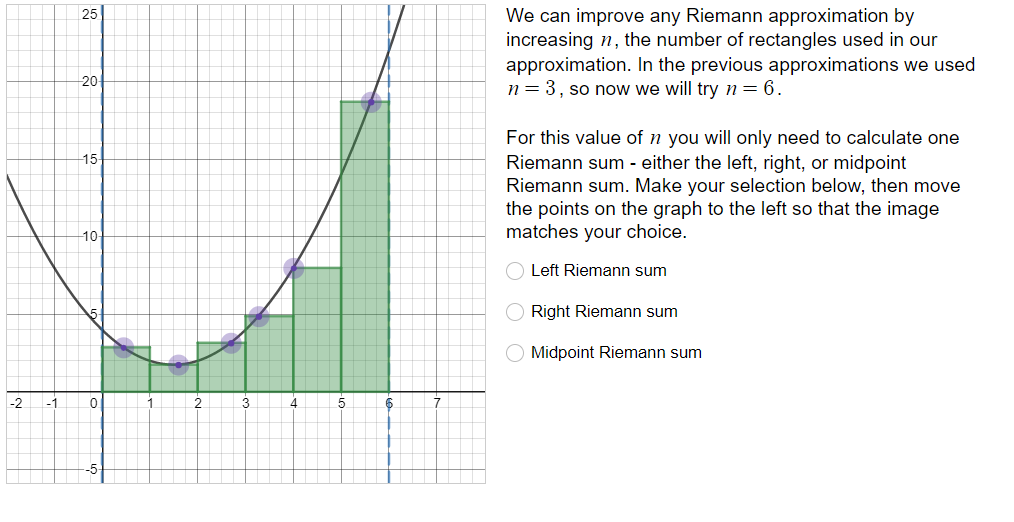# We can improve any Riemann approximation byincreasing n, the number of rectangles used in ourapproximation. In the previous approximations we usedn- 3, so now we will try n 62520For this value of n you will only need to calculate oneRiemann sum - either the left, right, or midpointRiemann sum. Make your selection below, then movethe points on the graph to the left so that the imagematches your choice1510Left Riemann sumRight Riemann sunmMidpoint Riemann sum2 -10

Question
37 viewshelp_outlineImage TranscriptioncloseWe can improve any Riemann approximation by increasing n, the number of rectangles used in our approximation. In the previous approximations we used n- 3, so now we will try n 6 25 20 For this value of n you will only need to calculate one Riemann sum - either the left, right, or midpoint Riemann sum. Make your selection below, then move the points on the graph to the left so that the image matches your choice 15 10 Left Riemann sum Right Riemann sunm Midpoint Riemann sum 2 -10 fullscreen
check_circle

Explanation:

Yes. Functions that increase on the interval [a,b] will be underestimated by left-hand Riemann sums and overestimated by right-hand Riemann sums. Decreasing functions have the reverse as true.

The midpoint Riemann sums is an attempt to balance these two extremes, so generally it is more accurate.

The Mean Value Theorem for Integrals guarantees (for appropriate functions f) that a point c exists in [a,b] such that the area under the curve is equal to the area f(c)⋅(b−a).

We can extend this idea to each subinterval in a Riemann sum. Each subinterval ii has a point c_i where the rectangular area matches the area under the function on the subinterval.

There likely isn't a nice pattern for identifying these points, however. The midpoint Riemann sum is usually the best easy approximation for these points.

Final Statement:

The option midpoint riemann sum is better approach.

...

### Want to see the full answer?

See Solution

#### Want to see this answer and more?

Solutions are written by subject experts who are available 24/7. Questions are typically answered within 1 hour.*

See Solution
*Response times may vary by subject and question.
Tagged in
MathCalculus

### Integration